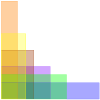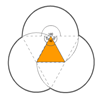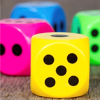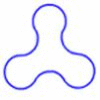# Resources tagged with: Curious

Filter by: Content type:
Age range:
Challenge level:

### There are 68 results

Broad Topics > Habits of Mind > Curious### Where to Land

##### Age 14 to 16 Challenge Level:

Chris is enjoying a swim but needs to get back for lunch. If she can swim at 3 m/s and run at 7m/sec, how far along the bank should she land in order to get back as quickly as possible?### Triangle Midpoints

##### Age 14 to 16 Challenge Level:

You are only given the three midpoints of the sides of a triangle. How can you construct the original triangle?### Think of Two Numbers

##### Age 11 to 14 Challenge Level:

Think of two whole numbers under 10, and follow the steps. I can work out both your numbers very quickly. How?### Curvy Areas

##### Age 14 to 16 Challenge Level:

Have a go at creating these images based on circles. What do you notice about the areas of the different sections?### Pick's Theorem

##### Age 14 to 16 Challenge Level:

Polygons drawn on square dotty paper have dots on their perimeter (p) and often internal (i) ones as well. Find a relationship between p, i and the area of the polygons.### Blue and White

##### Age 11 to 14 Challenge Level:

Identical squares of side one unit contain some circles shaded blue. In which of the four examples is the shaded area greatest?### Can They Be Equal?

##### Age 11 to 14 Challenge Level:

Can you find rectangles where the value of the area is the same as the value of the perimeter?### On the Edge

##### Age 11 to 14 Challenge Level:

If you move the tiles around, can you make squares with different coloured edges?### Perimeter Possibilities

##### Age 11 to 14 Challenge Level:

I'm thinking of a rectangle with an area of 24. What could its perimeter be?### Fit for Photocopying

##### Age 14 to 16 Challenge Level:

Explore the relationships between different paper sizes.### Hexy-metry

##### Age 14 to 16 Challenge Level:

A hexagon, with sides alternately a and b units in length, is inscribed in a circle. How big is the radius of the circle?### Marbles in a Box

##### Age 11 to 16 Challenge Level:

How many winning lines can you make in a three-dimensional version of noughts and crosses?### Trapezium Four

##### Age 14 to 16 Challenge Level:

The diagonals of a trapezium divide it into four parts. Can you create a trapezium where three of those parts are equal in area?### Partly Painted Cube

##### Age 14 to 16 Challenge Level:

Jo made a cube from some smaller cubes, painted some of the faces of the large cube, and then took it apart again. 45 small cubes had no paint on them at all. How many small cubes did Jo use?##### Age 11 to 14 Challenge Level:

Think of a number and follow my instructions. Tell me your answer, and I'll tell you what you started with! Can you explain how I know?### Largest Product

##### Age 11 to 14 Challenge Level:

Which set of numbers that add to 10 have the largest product?### More Number Pyramids

##### Age 11 to 14 Challenge Level:

When number pyramids have a sequence on the bottom layer, some interesting patterns emerge...### Opposite Vertices

##### Age 11 to 14 Challenge Level:

Can you recreate squares and rhombuses if you are only given a side or a diagonal?### Take Three from Five

##### Age 14 to 16 Challenge Level:

Caroline and James pick sets of five numbers. Charlie chooses three of them that add together to make a multiple of three. Can they stop him?### Searching for Mean(ing)

##### Age 11 to 16 Challenge Level:

If you have a large supply of 3kg and 8kg weights, how many of each would you need for the average (mean) of the weights to be 6kg?### Cuboid Challenge

##### Age 11 to 16 Challenge Level:

What's the largest volume of box you can make from a square of paper?### How Much Can We Spend?

##### Age 11 to 14 Challenge Level:

A country has decided to have just two different coins, 3z and 5z coins. Which totals can be made? Is there a largest total that cannot be made? How do you know?### What Numbers Can We Make?

##### Age 11 to 14 Challenge Level:

Imagine we have four bags containing a large number of 1s, 4s, 7s and 10s. What numbers can we make?### Beelines

##### Age 14 to 16 Challenge Level:

Is there a relationship between the coordinates of the endpoints of a line and the number of grid squares it crosses?### Number Pyramids

##### Age 11 to 14 Challenge Level:

Try entering different sets of numbers in the number pyramids. How does the total at the top change?### Litov's Mean Value Theorem

##### Age 11 to 14 Challenge Level:

Start with two numbers and generate a sequence where the next number is the mean of the last two numbers...### Special Numbers

##### Age 11 to 14 Challenge Level:

My two digit number is special because adding the sum of its digits to the product of its digits gives me my original number. What could my number be?### What's Possible?

##### Age 14 to 16 Challenge Level:

Many numbers can be expressed as the difference of two perfect squares. What do you notice about the numbers you CANNOT make?### Pair Products

##### Age 14 to 16 Challenge Level:

Choose four consecutive whole numbers. Multiply the first and last numbers together. Multiply the middle pair together. What do you notice?### What Numbers Can We Make Now?

##### Age 11 to 14 Challenge Level:

Imagine we have four bags containing numbers from a sequence. What numbers can we make now?### Summing Consecutive Numbers

##### Age 11 to 14 Challenge Level:

15 = 7 + 8 and 10 = 1 + 2 + 3 + 4. Can you say which numbers can be expressed as the sum of two or more consecutive integers?### Arithmagons

##### Age 11 to 16 Challenge Level:

Can you find the values at the vertices when you know the values on the edges?### Multiplication Arithmagons

##### Age 14 to 16 Challenge Level:

Can you find the values at the vertices when you know the values on the edges of these multiplication arithmagons?### Elevenses

##### Age 11 to 14 Challenge Level:

How many pairs of numbers can you find that add up to a multiple of 11? Do you notice anything interesting about your results?### Right Angles

##### Age 11 to 14 Challenge Level:

Can you make a right-angled triangle on this peg-board by joining up three points round the edge?### What's it Worth?

##### Age 11 to 16 Challenge Level:

There are lots of different methods to find out what the shapes are worth - how many can you find?### A Little Light Thinking

##### Age 14 to 16 Challenge Level:

Here is a machine with four coloured lights. Can you make two lights switch on at once? Three lights? All four lights?### Shifting Times Tables

##### Age 11 to 14 Challenge Level:

Can you find a way to identify times tables after they have been shifted up or down?### How Old Am I?

##### Age 14 to 16 Challenge Level:

In 15 years' time my age will be the square of my age 15 years ago. Can you work out my age, and when I had other special birthdays?### Square Coordinates

##### Age 11 to 14 Challenge Level:

A tilted square is a square with no horizontal sides. Can you devise a general instruction for the construction of a square when you are given just one of its sides?### Charlie's Delightful Machine

##### Age 11 to 16 Challenge Level:

Here is a machine with four coloured lights. Can you develop a strategy to work out the rules controlling each light?### Cola Can

##### Age 11 to 14 Challenge Level:

An aluminium can contains 330 ml of cola. If the can's diameter is 6 cm what is the can's height?##### Age 14 to 16 Challenge Level:

Explore when it is possible to construct a circle which just touches all four sides of a quadrilateral.### Semi-regular Tessellations

##### Age 11 to 16 Challenge Level:

Semi-regular tessellations combine two or more different regular polygons to fill the plane. Can you find all the semi-regular tessellations?### Satisfying Statements

##### Age 11 to 14 Challenge Level:

Can you find any two-digit numbers that satisfy all of these statements?### Which Solids Can We Make?

##### Age 11 to 14 Challenge Level:

Interior angles can help us to work out which polygons will tessellate. Can we use similar ideas to predict which polygons combine to create semi-regular solids?### Triangles and Petals

##### Age 14 to 16 Challenge Level:

An equilateral triangle rotates around regular polygons and produces an outline like a flower. What are the perimeters of the different flowers?### Cosy Corner

##### Age 11 to 14 Challenge Level:

Six balls are shaken. You win if at least one red ball ends in a corner. What is the probability of winning?### Dicey Operations

##### Age 11 to 14 Challenge Level:

Who said that adding, subtracting, multiplying and dividing couldn't be fun?### Arclets

##### Age 14 to 16 Challenge Level:

Each of the following shapes is made from arcs of a circle of radius r. What is the perimeter of a shape with 3, 4, 5 and n "nodes".